# Bernoulli equation

(diff) ← Older revision | Latest revision (diff) | Newer revision → (diff)

An ordinary first-order differential equation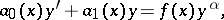whereis a real number other than zero or one. This equation was first studied by J. Bernoulli . The substitution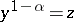converts the Bernoulli equation to a linear inhomogeneous first-order equation, . If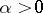, the solution of the Bernoulli equation is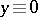; if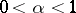, at some points the solution is no longer single-valued. Equations of the typeare also Bernoulli equations ifis considered as the independent variable, whileis an unknown function of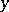.

How to Cite This Entry:
Bernoulli equation. Encyclopedia of Mathematics. URL: http://encyclopediaofmath.org/index.php?title=Bernoulli_equation&oldid=40764
This article was adapted from an original article by N.Kh. Rozov (originator), which appeared in Encyclopedia of Mathematics - ISBN 1402006098. See original article